# Boundary-Layer Theory Notes | Study Fluid Mechanics - Civil Engineering (CE)

## Civil Engineering (CE): Boundary-Layer Theory Notes | Study Fluid Mechanics - Civil Engineering (CE)

The document Boundary-Layer Theory Notes | Study Fluid Mechanics - Civil Engineering (CE) is a part of the Civil Engineering (CE) Course Fluid Mechanics.
All you need of Civil Engineering (CE) at this link: Civil Engineering (CE)

Introduction

When a real fluid flows over a solid body, the velocity of the fluid at the boundary will be zero. If the boundary is stationary. As we move away from the boundary in perpendicular direction velocity increases to the free stream velocity. It means velocity gradient (du/dy) will exist.

Note: Velocity gradient du/dy does not exist outside the boundary layer as outside the boundary layer velocity is constant and equal to free stream velocity.

Development of Boundary Layer

Development of boundary layer can be divided into three regions: laminar, transition, turbulent.
Reynolds number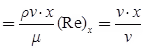For the laminar boundary layer
(Re)x < 5 × 105 (For flat plate) and if (Re)x > 5 × 105
where Re = Reynolds's number
Then, flow is turbulent.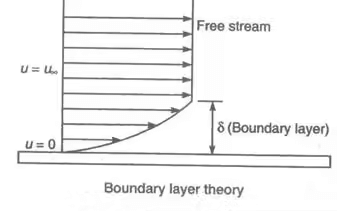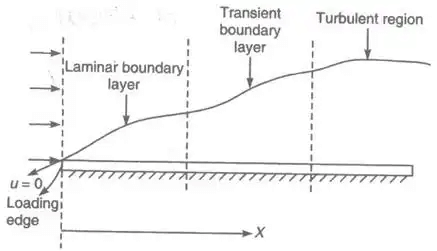Here, x is the distance from the leading edge in the horizontal direction.

Boundary-Layer Thickness (δ)

It is the distance from the boundary to the point where the velocity of the fluid is approximately equal to 99% of free stream velocity. It is represented by δ.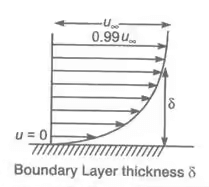Displacement Thickness (δ*)

It is observed that inside the boundary layer velocity of the fluid is less than the free stream velocity hence, discharge is less in this region. To compensate for the reduction in discharge the boundary is displaced outward in a perpendicular direction by some distance. This distance is called displacement thickness (δ*).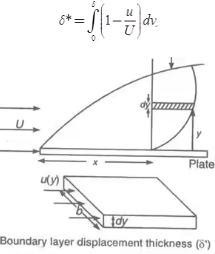Momentum Thickness (θ)

As due to boundary layer reduction in velocity occurs so, momentum also decreases. Momentum thickness is defined as the distance measured normal to the boundary of the solid body by which the boundary should be displaced to compensate for the reduction in the momentum of flowing fluid.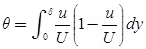Energy Thickness (δ**)

It is defined as distance measured perpendicular to the boundary of a solid body by which the boundary should be displaced to compensate for the reduction in kinetic energy of flowing fluid (KE decreases due to formation of the boundary layer)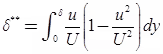Boundary Conditions for the Velocity Profile

Boundary conditions are as:
(a) At y = 0, u = 0, du / dy ≠ 0
(b) At y = δ, u = U, du / dy = 0

Laminar Flow

A flow in which fluid flows in layer and no intermixing with each other is known as laminar flow. For circular pipe, the flow will be laminar.

If Re = ρvD/μ < 2000
Where, ρ = Density of fluid, v = Velocity of fluid,
D = Diameter of pipe, μ = Viscosity of fluid.
For a flat plate, the flow will be laminar.
If Re = ρvL/μ < 5 x 105
Where L is the length of the plate.

Turbulent Flow

In this flow, an adjacent layer of fluid cross each other (particles of fluid move randomly instead of moving in a streamlined path), for flow inside the pipe. If Re > 4000, the flow is considered turbulent, for flat plate, Re > 5 × 105.
Von Karman Momentum Integral Equation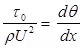where, θ = momentum thickness
Shear stress: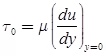Where, U = Free stream velocity; ρ = Density of fluid.
Local Coefficient of Drag (CD*)

It is defined as the ratio of the shear stress τ0 to the quantity1/2ρU2
It is denoted by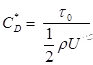Average Coefficient of Drag (CD):

It is defined as the ratio of the total drag force to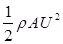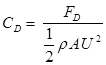Where, A = Area of surface,
U = Free stream velocity, ρ = Mass density of the fluid.

Blasius Experiment Results

For laminar flow,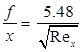Coefficient of drag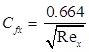Average coefficient of drag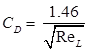For turbulent flow,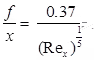where x = Distance from leading-edge, Rex = Reynolds's number for length x.
ReL = Reynolds's number at end of the plate
Coefficient of drag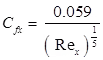Average coefficient of drag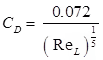For laminar flow
f ∝ √x
f = Boundary layer thickness,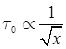τ0 = Shear stress at the solid surface
x = Distance from where solid surface starts.
Velocity profile for turbulent boundary layer is
u / U = (9y / f)1 / n ⇒ 1 / n = 1 / τ
⇒ 5 x 105 < Re < 107

Conditions for Boundary Layer Separation: Let us take curve surface ABCSD where fluid flow separation print S is determined from the condition (du/dy)y = 0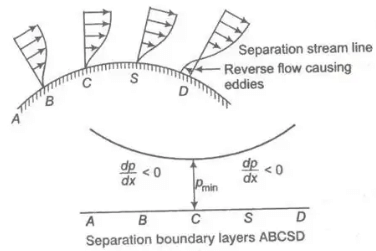If (du / dy)y = 0 = -ve,

the flow is separated (dp / dx > 0)

If (du / dy)y = 0 = 0,

the flow is on the average of separation (∂ρ / ∂x = o)

If (∂u / ∂y) y = 0 = +ve the flow will not separate or flow will remain attained (dp / dx < 0)

Methods of Preventing Separation of Boundary Layer

Suction of slow-moving fluid by a suction slot.

• Supplying additional energy from a blower.
• Providing a bypass in the slotted
• Rotating boundary in the direction of flow.
• Providing small divergence in a diffuser.
• Providing guide blades in a bend.
• Providing a tripwire ring in the laminar region for the flow over a sphere.
The document Boundary-Layer Theory Notes | Study Fluid Mechanics - Civil Engineering (CE) is a part of the Civil Engineering (CE) Course Fluid Mechanics.
All you need of Civil Engineering (CE) at this link: Civil Engineering (CE)Use Code STAYHOME200 and get INR 200 additional OFF

## Fluid Mechanics

43 videos|72 docs|72 tests

Track your progress, build streaks, highlight & save important lessons and more!

,

,

,

,

,

,

,

,

,

,

,

,

,

,

,

,

,

,

,

,

,

;